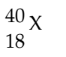# Problem: Determine the number of protons, neutrons and electrons in the following: A) p+= 18 n° = 22 e- = 40 B) p+= 40 n° = 22 e- = 18 C) p+= 18 n° = 22 e- = 18 D) p+= 18 n° = 18 e- = 2 E) p+= 22 n° = 18 e- = 18

###### FREE Expert Solution
83% (134 ratings)
###### Problem Details

Determine the number of protons, neutrons and electrons in the following:

A) p+= 18 n° = 22 e- = 40

B) p+= 40 n° = 22 e- = 18

C) p+= 18 n° = 22 e- = 18

D) p+= 18 n° = 18 e- = 2

E) p+= 22 n° = 18 e- = 18